Published

# One Handed Keyboard

This project is a one handed keyboard that is intended to reduce the movement required to type as well as increase the typing speed.

IntermediateFull instructions provided20 hours1,187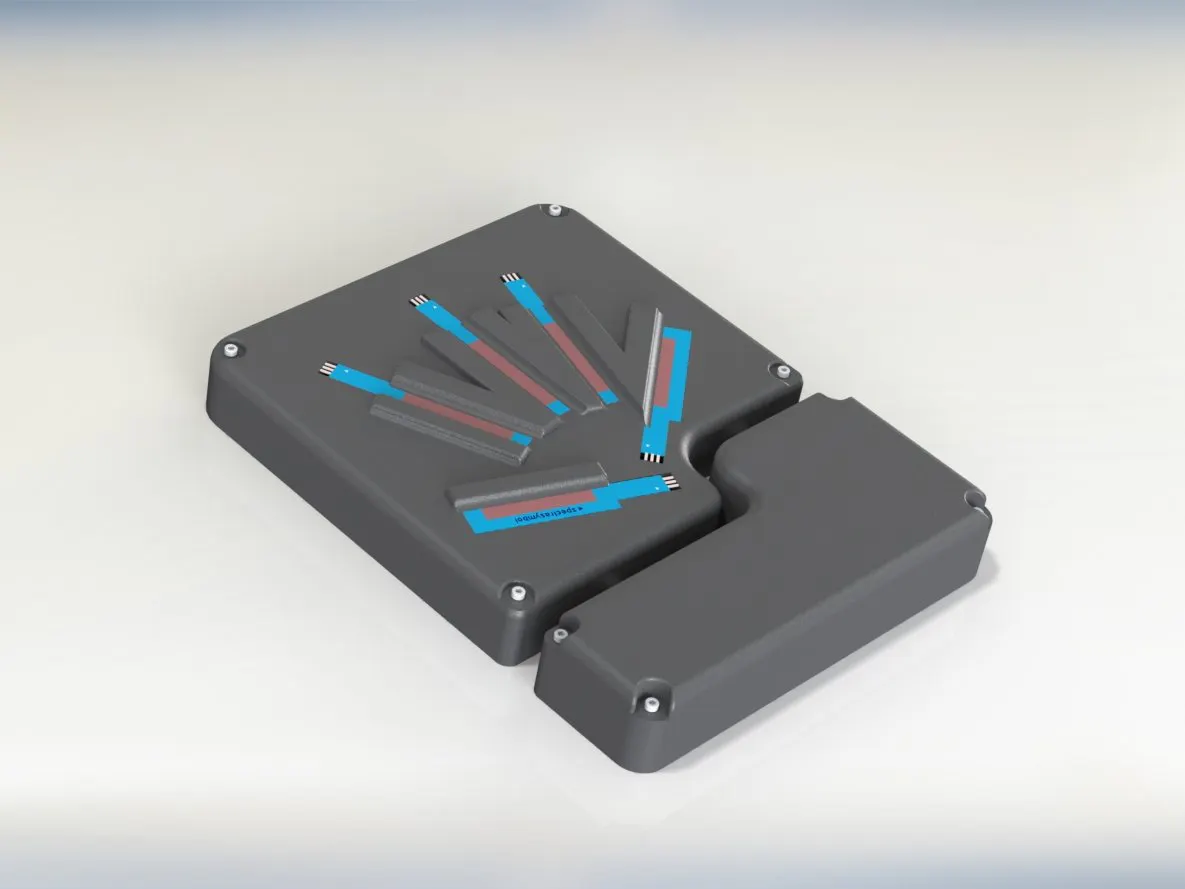## Things used in this project

### Hardware components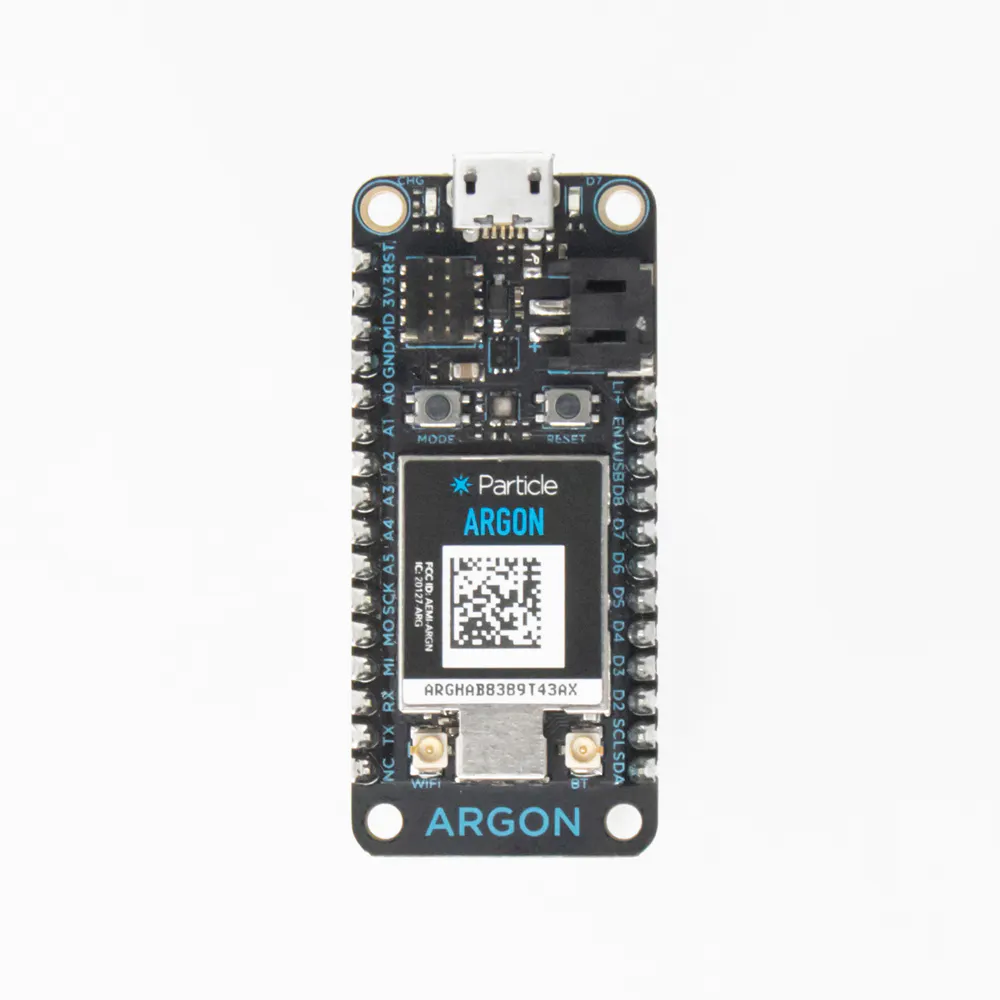Particle Argon
×3Resistor 10k ohm
×5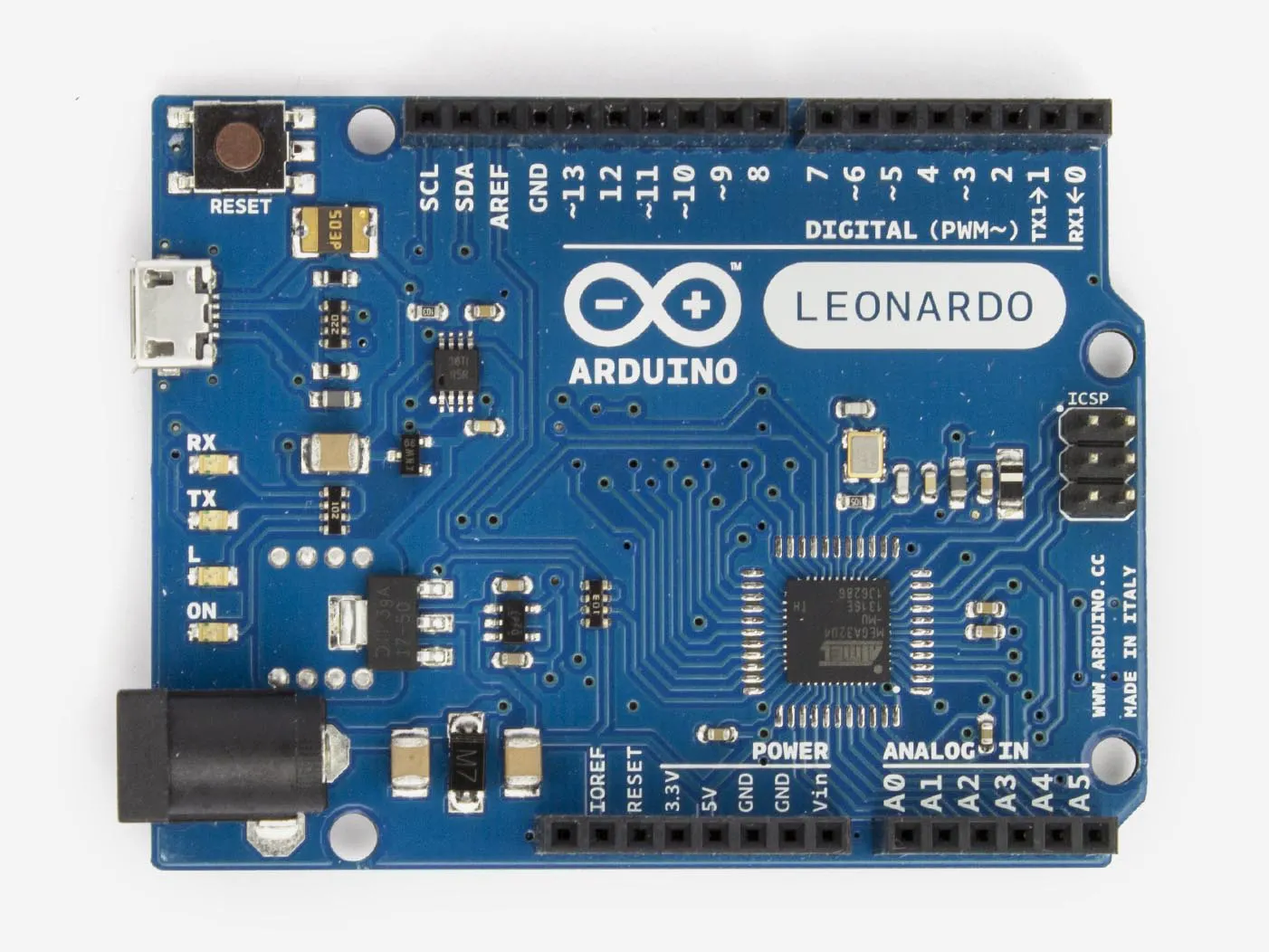Arduino Leonardo
×1
 Printed Circuit Board
×1
 Power Supply Module 1PC
×1
 Power Converter
×1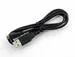USB-A to Micro-USB Cable
×1
 Wiring
×1
 3-D Printed Housing
×1
 Potentiometer
×5
×18
 Pins w/E-Clip
×1

### Software apps and online services

 Solidworks
 build.particle.io
 Fritzing
 create.arduino
 ThingSpeak
 Inkscape
 Bantam Tools

### Hand tools and fabrication machinesSoldering iron (generic)
 Allen Wrench Set
 3-D Printer
 Bantam Tools Desktop PCB Milling Machine
 Wire Cutters/Strippers

## Schematics

### Fritzing Diagram

Wire Diagram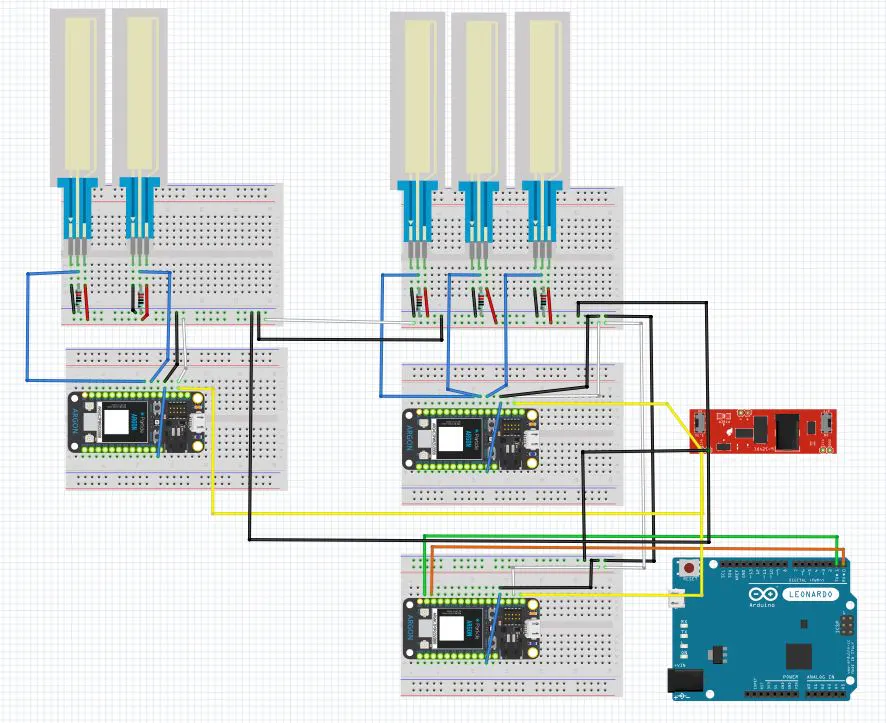### .brb Circuit

This circuit diagram is constructed using EagleCad

## Code

### Main Argon

C/C++
This code is uploaded to the Argon that is connected to the Arduino
```void myHandler(const char *event, const char *data) {

String responce = "0";

if (strcmp(data, "0")==0) {}
else if (strcmp(data, "1")==0){
Serial1.write(1);
responce = "10";    }
else if (strcmp(data, "2")==0){
Serial1.write(2);
responce = "10";    }
else if (strcmp(data, "3")==0){
Serial1.write(3);
responce = "10";    }
else if (strcmp(data, "4")==0){
Serial1.write(4);
responce = "10";    }
else if (strcmp(data, "5")==0){
Serial1.write(5);
responce = "10";    }
else if (strcmp(data, "6")==0){
Serial1.write(6);
responce = "10";    }
else if (strcmp(data, "7")==0){
Serial1.write(7);
responce = "10";    }
else if (strcmp(data, "8")==0){
Serial1.write(8);
responce = "10";    }
else if (strcmp(data, "9")==0){
Serial1.write(9);
responce = "10";    }
else if (strcmp(data, "10")==0){
Serial1.write(10);
responce = "10";    }
else if (strcmp(data, "11")==0){
Serial1.write(11);
responce = "10";    }
else if (strcmp(data, "12")==0){
Serial1.write(12);
responce = "10";    }
else if (strcmp(data, "13")==0){
Serial1.write(13);
responce = "10";    }
else if (strcmp(data, "14")==0){
Serial1.write(14);
responce = "10";    }
else if (strcmp(data, "15")==0){
Serial1.write(15);
responce = "10";    }
else if (strcmp(data, "16")==0){
Serial1.write(16);
responce = "10";    }
else if (strcmp(data, "17")==0){
Serial1.write(17);
responce = "10";    }
else if (strcmp(data, "18")==0){
Serial1.write(18);
responce = "5";    }
else if (strcmp(data, "19")==0){
Serial1.write(19);
responce = "5";    }
else if (strcmp(data, "20")==0){
Serial1.write(20);
responce = "5";    }
else if (strcmp(data, "21")==0){
Serial1.write(21);
responce = "5";    }
else if (strcmp(data, "22")==0){
Serial1.write(22);
responce = "5";    }
else if (strcmp(data, "23")==0){
Serial1.write(23);
responce = "5";    }
else if (strcmp(data, "24")==0){
Serial1.write(24);
responce = "5";    }
else if (strcmp(data, "25")==0){
Serial1.write(25);
responce = "5";    }
else if (strcmp(data, "26")==0){
Serial1.write(26);
responce = "5";    }
else if (strcmp(data, "27")==0){
Serial1.write(27);
responce = "5";    }
else if (strcmp(data, "28")==0){
Serial1.write(28);
responce = "5";    }
else if (strcmp(data, "29")==0){
Serial1.write(29);
responce = "5";    }
else if (strcmp(data, "30")==0){
Serial1.write(30);
responce = "5";    }
else {}

Particle.publish("myresponce", responce, PRIVATE);
delay(1000);

}

void setup() {

Serial1.begin(9600);

}

void loop() {

Particle.subscribe("Key_Stroke", myHandler, MY_DEVICES);
delay(1000);

}
```

### Argon One

C/C++
This code is uploaded to the Argon that has three softpots
```void myHandler(const char *event, const char *data) {

if (strcmp(data, "10")==0){
digitalWrite(D7, HIGH);
delay(500);
digitalWrite(D7, LOW);
}
else {

}

}

void setup() {

pinMode(A1, INPUT); // Thumb Finger
pinMode(A2, INPUT); // Index Finger
pinMode(A3, INPUT); // Middle Finger
pinMode(D7, OUTPUT);

}

int arr = {0};

void loop() {

int td = 10;
int t = 50;
int n = 8;
int i = 1;

delay(td);
int thumbfinger = analogRead(A1); // 0 - 4095 range
int thumb = map(thumbfinger, 0, 4095, 0, 7); // 0 - 7 range

delay(td);
int indexfinger = analogRead(A2); // 0 - 4095 range
int index = map(indexfinger, 0, 4095, 0, 7); // 0 - 7 range

delay(td);
int middlefinger = analogRead(A3); // 0 - 4095 range
int middle = map(middlefinger, 0, 4095, 0, 7); // 0 - 7 range

String Data = String(0);

while (i < 19){
if (arr[i]>16){
memset(arr, 0, sizeof(arr));
}
i++;
}

switch(thumb) {
case 0:
Data = String(0);
break;
case 1:
arr++;
if (arr > n+8){
memset(arr, 0, sizeof(arr));
Data = String(1);
Particle.publish("Key_Stroke", Data, PRIVATE);
delay(1000);
delay(t);
}
break;
case 2:
arr++;
if (arr > n+3){
memset(arr, 0, sizeof(arr));
Data = String(2);
Particle.publish("Key_Stroke", Data, PRIVATE);
delay(1000);
delay(t);
}
break;
case 3:
arr++;
if (arr > n){
memset(arr, 0, sizeof(arr));
Data = String(3);
Particle.publish("Key_Stroke", Data, PRIVATE);
delay(1000);
delay(t);
}
break;
case 4:
arr++;
if (arr > n){
memset(arr, 0, sizeof(arr));
Data = String(4);
Particle.publish("Key_Stroke", Data, PRIVATE);
delay(1000);
delay(t);
}
break;
case 5:
arr++;
if (arr > n){
memset(arr, 0, sizeof(arr));
Data = String(5);
Particle.publish("Key_Stroke", Data, PRIVATE);
delay(1000);
delay(t);
}
break;
case 6:
arr++;
if (arr > n){
memset(arr, 0, sizeof(arr));
Data = String(6);
Particle.publish("Key_Stroke", Data, PRIVATE);
delay(1000);
delay(t);
}
break;
default:
Data = String(0);
break;
}

switch(index){
case 0:
Data = String(0);
break;
case 1:
arr++;
if (arr > n+8){
memset(arr, 0, sizeof(arr));
Data = String(7);
Particle.publish("Key_Stroke", Data, PRIVATE);
delay(1000);
delay(t);
}
break;
case 2:
arr++;
if (arr > n+3){
memset(arr, 0, sizeof(arr));
Data = String(8);
Particle.publish("Key_Stroke", Data, PRIVATE);
delay(1000);
delay(t);
}
break;
case 3:
arr++;
if (arr > n){
memset(arr, 0, sizeof(arr));
Data = String(9);
Particle.publish("Key_Stroke", Data, PRIVATE);
delay(1000);
delay(t);
}
break;
case 4:
arr++;
if (arr > n){
memset(arr, 0, sizeof(arr));
Data = String(10);
Particle.publish("Key_Stroke", Data, PRIVATE);
delay(1000);
delay(t);
}
break;
case 5:
arr++;
if (arr > n){
memset(arr, 0, sizeof(arr));
Data = String(11);
Particle.publish("Key_Stroke", Data, PRIVATE);
delay(1000);
delay(t);
}
break;
case 6:
arr++;
if (arr > n){
memset(arr, 0, sizeof(arr));
Data = String(12);
Particle.publish("Key_Stroke", Data, PRIVATE);
delay(1000);
delay(t);
}
break;
default:
Data = String(0);
break;
}

switch(middle) {
case 0:
Data = String(0);
break;
case 1:
arr++;
if (arr > n+8){
memset(arr, 0, sizeof(arr));
Data = String(13);
Particle.publish("Key_Stroke", Data, PRIVATE);
delay(1000);
delay(t);
}
break;
case 2:
arr++;
if (arr > n+3){
memset(arr, 0, sizeof(arr));
Data = String(14);
Particle.publish("Key_Stroke", Data, PRIVATE);
delay(1000);
delay(t);
}
break;
case 3:
arr++;
if (arr > n){
memset(arr, 0, sizeof(arr));
Data = String(15);
Particle.publish("Key_Stroke", Data, PRIVATE);
delay(1000);
delay(t);
}
break;
case 4:
arr++;
if (arr > n){
memset(arr, 0, sizeof(arr));
Data = String(16);
delay(t);
}
break;
case 5:
arr++;
if (arr > n){
memset(arr, 0, sizeof(arr));
Data = String(17);
delay(t);
}
break;
case 6:
arr++;
if (arr > n){
memset(arr, 0, sizeof(arr));
Data = String(18);
Particle.publish("Key_Stroke", Data, PRIVATE);
delay(1000);
delay(t);
}
break;
default:
Data = String(0);
break;
}

Particle.subscribe("res", myHandler, MY_DEVICES);

}
```

### Argon Two

C/C++
This code is uploaded to the Argon with two softpots
```void myHandler(const char *event, const char *data) {

if (strcmp(data, "5")==0){
digitalWrite(D7, HIGH);
delay(500);
digitalWrite(D7, LOW);
}
else {

}

}

void setup() {

pinMode(A1, INPUT); // forth Finger
pinMode(A2, INPUT); // pinky Finger
pinMode(D7, OUTPUT);

}

int arr = {0};

void loop() {

int td = 10;
int t = 50;
int n = 8;
int i = 1;

delay(td);
int fourthfinger = analogRead(A1); // 0 - 4095 range
int fourth = map(fourthfinger, 0, 4095, 0, 7); // 0 - 7 range

delay(td);
int pinkyfinger = analogRead(A2); // 0 - 4095 range
int pinky = map(pinkyfinger, 0, 4095, 0, 7); // 0 - 7 range

String Data = String(0);

while (i < 19){
if (arr[i]>16){
memset(arr, 0, sizeof(arr));
}
i++;
}

switch(fourth) {
case 0:
Data = String (0);
break;
case 1:
arr++;
if (arr > n+8){
memset(arr, 0, sizeof(arr));
Data = String (19);
Particle.publish("Key_Stroke", Data, PRIVATE);
delay(1000);
delay(t);
}
break;
case 2:
arr++;
if (arr > n+3){
memset(arr, 0, sizeof(arr));
Data = String (20);
Particle.publish("Key_Stroke", Data, PRIVATE);
delay(1000);
delay(t);
}
break;
case 3:
arr++;
if (arr > n){
memset(arr, 0, sizeof(arr));
Data = String (21);
Particle.publish("Key_Stroke", Data, PRIVATE);
delay(1000);
delay(t);
}
break;
case 4:
arr++;
if (arr > n){
memset(arr, 0, sizeof(arr));
Data = String (22);
Particle.publish("Key_Stroke", Data, PRIVATE);
delay(1000);
delay(t);
}
break;
case 5:
arr++;
if (arr > n){
memset(arr, 0, sizeof(arr));
Data = String (23);
Particle.publish("Key_Stroke", Data, PRIVATE);
delay(1000);
delay(t);
}
break;
case 6:
arr++;
if (arr > n){
memset(arr, 0, sizeof(arr));
Data = String (24);
Particle.publish("Key_Stroke", Data, PRIVATE);
delay(1000);
delay(t);
}
break;
default:
Data = String (0);
break;
}

switch(pinky){
case 0:
Data = String (0);
break;
case 1:
arr++;
if (arr > n+8){
memset(arr, 0, sizeof(arr));
Data = String (25);
Particle.publish("Key_Stroke", Data, PRIVATE);
delay(1000);
delay(t);
}
break;
case 2:
arr++;
if (arr > n+3){
memset(arr, 0, sizeof(arr));
Data = String (26);
Particle.publish("Key_Stroke", Data, PRIVATE);
delay(1000);
delay(t);
}
break;
case 3:
arr++;
if (arr > n){
memset(arr, 0, sizeof(arr));
Data = String (27);
Particle.publish("Key_Stroke", Data, PRIVATE);
delay(1000);
delay(t);
}
break;
case 4:
arr++;
if (arr > n){
memset(arr, 0, sizeof(arr));
Data = String (28);
Particle.publish("Key_Stroke", Data, PRIVATE);
delay(1000);
delay(t);
}
break;
case 5:
arr++;
if (arr > n){
memset(arr, 0, sizeof(arr));
Data = String (29);Particle.publish("Key_Stroke", Data, PRIVATE);
delay(1000);
delay(t);
}
break;
case 6:
arr++;
if (arr > n){
memset(arr, 0, sizeof(arr));
Data = String (30);
Particle.publish("Key_Stroke", Data, PRIVATE);
delay(1000);
delay(t);
}
break;
default:
Data = String (0);
break;
}

Particle.subscribe("res", myHandler, MY_DEVICES);

}
```

### Arduino

C/C++
This code is to be uploaded to the Arduino that is connected to the computer.
```#include <Keyboard.h>

char enterKey = KEY_LEFT_CTRL;
char backspaceKey = KEY_BACKSPACE;
char shiftKey = KEY_RIGHT_SHIFT;

void setup() {
Serial1.begin(9600);
Keyboard.begin();
}

void loop() {

int t = 50;
int n = 4;

case 0:
break;
case 1:
Keyboard.press(backspaceKey);
Keyboard.release(backspaceKey);
break;
case 2:
Keyboard.print(" ");
break;
case 3:
Keyboard.press(enterKey);
Keyboard.release(enterKey);
break;
case 4:
Keyboard.press('x');
Keyboard.releaseAll();
break;
case 5:
Keyboard.press('y');
Keyboard.releaseAll();
break;
case 6:
Keyboard.press('z');
Keyboard.releaseAll();
break;
case 7:
Keyboard.press('a');
Keyboard.releaseAll();
break;
case 8:
Keyboard.press('b');
Keyboard.releaseAll();
break;
case 9:
Keyboard.press('c');
Keyboard.releaseAll();
break;
case 10:
Keyboard.press('d');
Keyboard.releaseAll();
break;
case 11:
Keyboard.press('e');
Keyboard.releaseAll();
break;
case 12:
Keyboard.press('f');
Keyboard.releaseAll();
break;
case 13:
Keyboard.press('g');
Keyboard.releaseAll();
break;
case 14:
Keyboard.press('h');
Keyboard.releaseAll();
break;
case 15:
Keyboard.press('i');
Keyboard.releaseAll();
break;
case 16:
Keyboard.press('j');
Keyboard.releaseAll();
break;
case 17:
Keyboard.press('k');
Keyboard.releaseAll();
break;
case 18:
Keyboard.press('l');
Keyboard.releaseAll();
break;
case 19:
Keyboard.press('m');
Keyboard.releaseAll();
break;
case 20:
Keyboard.press('n');
Keyboard.releaseAll();
break;
case 21:
Keyboard.press('o');
Keyboard.releaseAll();
break;
case 22:
Keyboard.press('p');
Keyboard.releaseAll();
break;
case 23:
Keyboard.press('q');
Keyboard.releaseAll();
break;
case 24:
Keyboard.press('r');
Keyboard.releaseAll();
break;
case 25:
Keyboard.press('s');
Keyboard.releaseAll();
break;
case 26:
Keyboard.press('t');
Keyboard.releaseAll();
break;
case 27:
Keyboard.press('u');
Keyboard.releaseAll();
break;
case 28:
Keyboard.press('v');
Keyboard.releaseAll();
break;
case 29:
Keyboard.press('w');
Keyboard.releaseAll();
break;
case 30:
Keyboard.press(shiftKey);
Keyboard.release(shiftKey);
break;
default:
break;
}
}
```

## Credits

### Avery Turley

1 project • 1 follower

### Josh Liddle

1 project • 0 followers

### Craig Williams

1 project • 0 followers